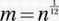# SAT Math Multiple Choice Question 622: Answer and Explanation

### Test Information

Question: 622

7. If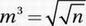, where n > 0, what is the value of m in terms of n?

• A.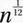• B.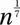• C.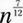• D.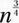Explanation:

A

For this question, we need to know two Laws of Exponentials from Chapter 9: Law #8 and Law #9. First, we use Law #9 to translate the radicals into exponents.

Given equation: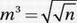Apply Law of Exponentials #9: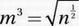Apply Law of Exponentials #9 again: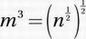Apply Law of Exponentials #8: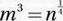Raise to the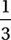power: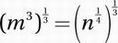Apply Law of Exponentials #8 again: